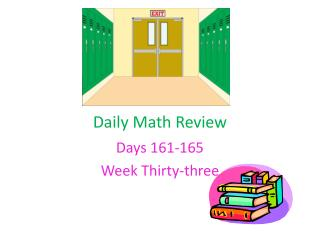DownloadDownload PresentationDaily Math Review

# Daily Math Review

Download Presentation## Daily Math Review

- - - - - - - - - - - - - - - - - - - - - - - - - - - E N D - - - - - - - - - - - - - - - - - - - - - - - - - - -
##### Presentation Transcript

1. Daily Math Review Days 161-165 Week Thirty-three

2. Monday 1. Name the underlined place. 1,648,294 2. Give the value of the underlined place. 3,523,487 3. Round. 3,578,942 Subtract. 4. 1 7/8 – 2/3 5. 9 2/5– 4 5/8 6. 10 3/5– 6 7/10

3. Tuesday 1. 3 m = _____ mm 2. 6 m = _____ cm 3. 4 m = ______ cm 4. 4 km = _______ m 5. 2 g = ______ mg 6. 7 kg = ______ g The table below shows the amount of money raised by students during a bake sale. Student Amount Raised Emily \$32.75 Mindy \$14.25 Nelly \$21.50 Ryan \$18.25 Zander \$11.00 A. Which student raised the greatest amount of money? B. Give 2 possible reasons that could explain why different students raised different amounts of money.

4. Wednesday 1. Which property of whole numbers is demonstrated by the number sentence below? 2+ (43 x 3) = 0 +2 + (43 x 3) a. Identity property of addition b. Associative property of addition c. Identity property of multiplication d. Commutative property of addition 2. The 36 student’s in Mrs. Ponder’s class made 4 items to sell at the school yard sale. Each item will be sold for 6 dollars. Which number sentence below could be used to determine the total number of dollars that will be collected if every one of those items is sold? a. (36 X 4) + 6 = ____ b. (36 + 4) X 6 = ____ c. (36 X 4) X 6 = _____ d. (36 ÷ 4 ) x 5 = _____ 3. Which number sentence below demonstrates the associative property of addition? a. (34 + 16) + 24 = (16 + 34) + 24 b. 27 + 40 = ( 27 + 40) + 0 c. ( 71 + 11) + 30 = 71 + ( 11 + 30) d. 51 + 37 = 1 X ( 51 + 37)

5. Thursday Map of Water Valley Camp Resort Sun Lee and his family went to Water Valley Camp Resort for vacation. Write the coordinates for each place or figure on the map.